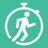# Time and DistanceQuantitative Aptitude Questions and Answers section on “Time and Distance” with solution and explanation for competitive examinations such as CAT, MBA, SSC, Bank PO, Bank Clerical and other examinations.

# A car travels at a speed of 60 km/hr and covers a particular distance in one hour. How long will it take for another car to cover the same distance at 40 km/hr?

A car travels at a speed of 60 km/hr and covers a particular distance in one hour. How long will it take for another car to cover the same distance at 40 km/hr? [A]$latex 1\ hours$ [B]$latex \frac{3}{2}\ hours&s=1$ [C]$latex 2\ hours$ [D]$latex \frac{5}{2}\ hours&s=1$ Show Answer $latex \mathbf{\frac{3}{2}\ hours}&s=1$ Distance = Speed $latex \times$ ..

# Motor-cyclist P started his journey at a speed of 30 km/hr. After 30 minutes, motor cyclist Q started from the same place but with the speed of 40 km/hr. How much time will Q take to overtake P?

Motor-cyclist P started his journey at a speed of 30 km/hr. After 30 minutes, motor cyclist Q started from the same place but with a speed of 40 km/hr. How much time will Q take to overtake P? [A]$latex 1\ hours$ [B]$latex \frac{3}{8}\ hours&s=1$ [C]$latex \frac{3}{2}\ hours&s=1$ [D]$latex 2\ hours$ Show Answer $latex \mathbf{\frac{3}{2}\ hours}&s=1$ ..

# Aptitude Question ID: 106308

A student goes to school at the rate of $latex \frac{5}{2}&s=1$ km/hr and reaches 6 minutes late. If he travels at the speed of 3 km/hr, he reaches 10 minutes earlier. The distance of the school is : [A]4 km [B]10 km [C]20 km [D]45 km Show Answer 4 km Distance of school = x ..